1624358712

# Graph Neural Networks - Expressive Power & Weisfeiler-Lehman Test

Traditional feed-forward networks (multi-layer perceptrons) are known to be universal approximators: they can approximate any smooth function to any desired accuracy. For graph neural networks, which have emerged relatively recently, the representation properties are less understood. It often happens to observe in experiments that graph neural networks excel on some datasets but at the same time perform disappointingly on others. In order to get to the root of this behaviour, one has to answer the question: how powerful are graph neural networks?

One of the challenges is that graphs encountered in applications are combinations of continuous and discrete structures (node- and edge features and connectivity, respectively), and thus this question can be posed in different ways.

#testing #expressive power #graph neural networks

## Buddha Community1624358712

## Graph Neural Networks - Expressive Power & Weisfeiler-Lehman Test

Traditional feed-forward networks (multi-layer perceptrons) are known to be universal approximators: they can approximate any smooth function to any desired accuracy. For graph neural networks, which have emerged relatively recently, the representation properties are less understood. It often happens to observe in experiments that graph neural networks excel on some datasets but at the same time perform disappointingly on others. In order to get to the root of this behaviour, one has to answer the question: how powerful are graph neural networks?

One of the challenges is that graphs encountered in applications are combinations of continuous and discrete structures (node- and edge features and connectivity, respectively), and thus this question can be posed in different ways.

#testing #expressive power #graph neural networks1594175520

## Expressive Power of Graph Neural Networks and The Weisfeiler

Do you have a feeling that deep learning on graphs is a bunch of heuristics that work sometimes and nobody has a clue why? In this post, I discuss the graph isomorphism problem, the Weisfeiler-Lehman heuristic for graph isomorphism testing, and how it can be used to analyse the expressive power of graph neural networks.

#express #graph networks #weisfeiler #programming1595691960

## How Graph Convolutional Networks (GCN) Work

In this post, we’re gonna take a close look at one of the well-known Graph neural networks named GCN. First, we’ll get the intuition to see how it works, then we’ll go deeper into the maths behind it.

## Why Graphs?

Many problems are graphs in true nature. In our world, we see many data are graphs, such as molecules, social networks, and paper citations networks.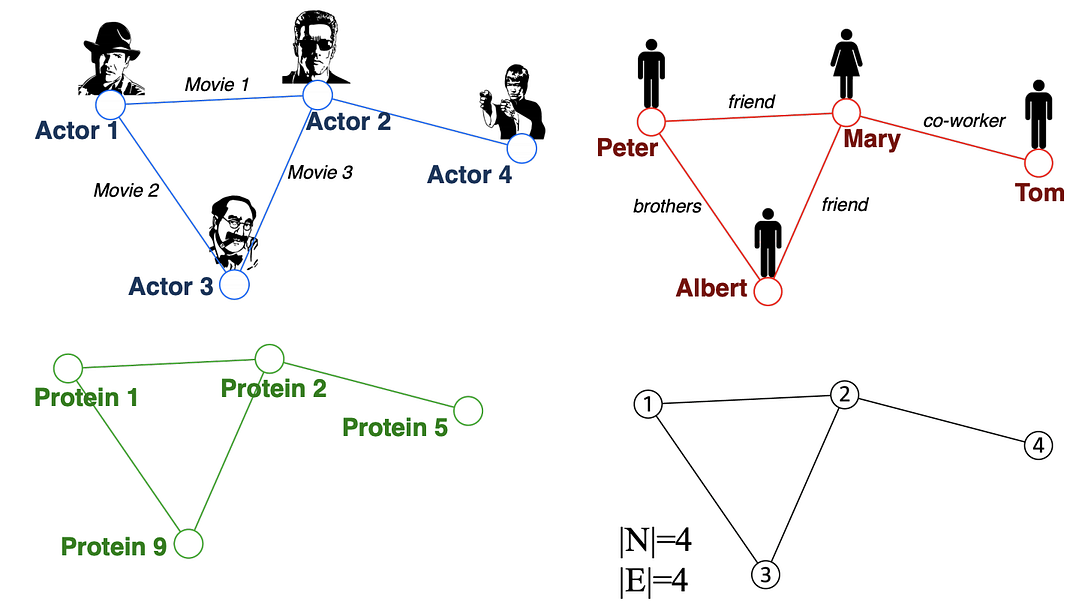Examples of graphs. (Picture from )

• Node classification: Predict a type of a given node
• Community detection: Identify densely linked clusters of nodes
• Network similarity: How similar are two (sub)networks

## Machine Learning Lifecycle

In the graph, we have node features (the data of nodes) and the structure of the graph (how nodes are connected).

For the former, we can easily get the data from each node. But when it comes to the structure, it is not trivial to extract useful information from it. For example, if 2 nodes are close to one another, should we treat them differently to other pairs? How about high and low degree nodes? In fact, each specific task can consume a lot of time and effort just for Feature Engineering, i.e., to distill the structure into our features.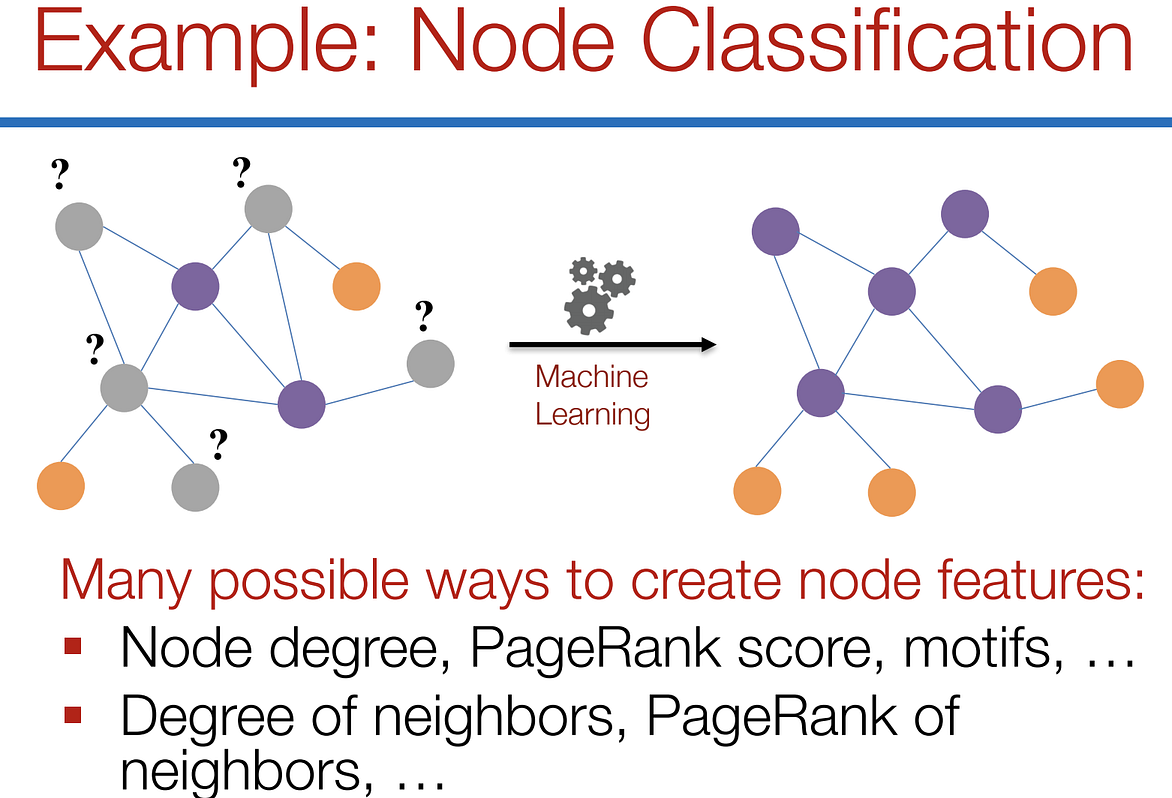Feature engineering on graphs. (Picture from )

It would be much better to somehow get both the node features and the structure as the input, and let the machine to figure out what information is useful by itself.

That’s why we need Graph Representation Learning.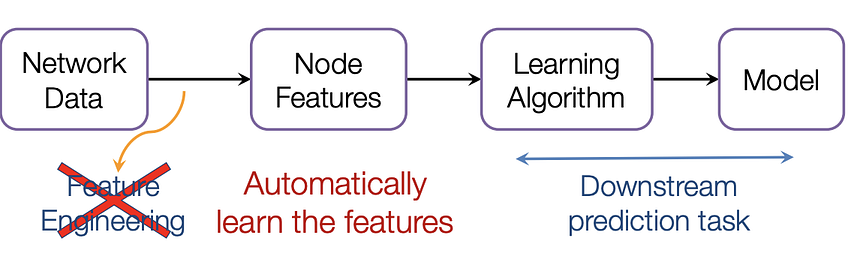We want the graph can learn the “feature engineering” by itself. (Picture from )

# Graph Convolutional Networks (GCNs)

Paper: Semi-supervised Classification with Graph Convolutional Networks(2017) 

GCN is a type of convolutional neural network that can work directly on graphs and take advantage of their structural information.

it solves the problem of classifying nodes (such as documents) in a graph (such as a citation network), where labels are only available for a small subset of nodes (semi-supervised learning).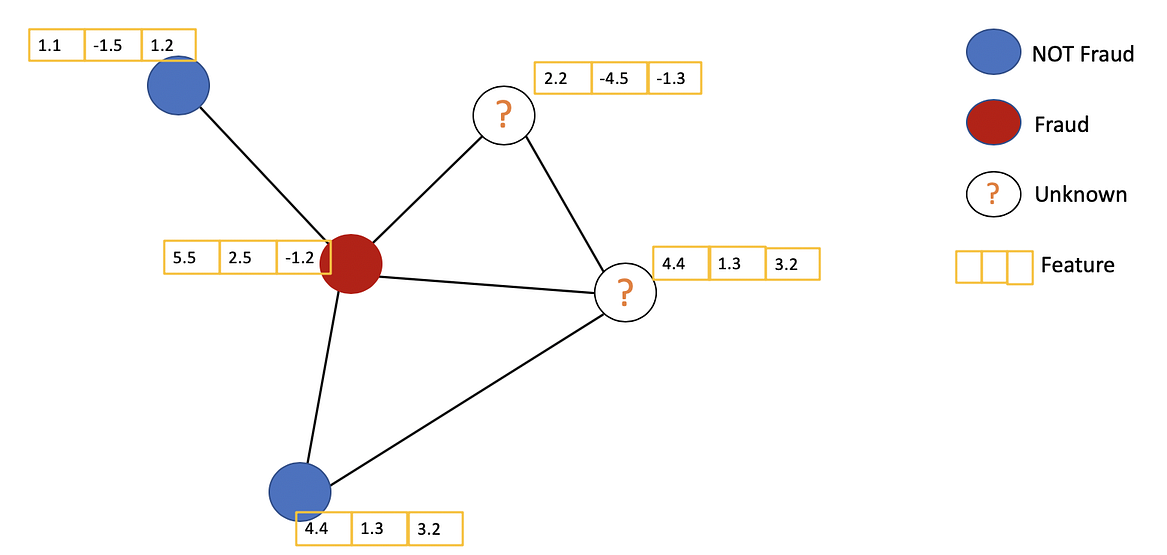Example of Semi-supervised learning on Graphs. Some nodes dont have labels (unknown nodes).

#graph-neural-networks #graph-convolution-network #deep-learning #neural-networks1602838800

## SimGNN: Similarity Computation via Graph Neural Networks

This post will summarize the paper SimGNN which aims for fast graph similarity computation. Graphs are structures that are used to link different entities that we call nodes using relationships called edges. Graphs exist everywhere from bonds between the atoms to friends on Facebook, all these scenarios can be represented as a graph. One of the fundamental graph problems includes finding similarity between graphs. The similarity between graphs can be defined using these metrics :

1. Graph Edit Distance
2. Maximum Common Subgraph

However, currently available algorithms that are used to calculate these metrics have high complexities and it is not yet possible to compute exact GED using these for graphs having more than 16 nodes.

Some ways to compute these metrics are :

1. Pruning verification Framework
2. Approximating the GED in fast and heuristic ways

SimGNN follows another approach to tackle this problem i.e turning similarity computation problem into a learning problem.

Before getting into how SimGNN works, we must know the requirements to be satisfied by this model. It includes :

1. Representation Invariant: Different representations of the same graph should give the same results.
2. **Inductive: **Should be able to predict results for unseen graphs.
3. Learnable: Must work on different similarity metrics like GED and MCS

**SimGNN Approach: **To achieve the above-stated requirements, SimGNN uses two strategies

1. Design Learnable Embedding Function: This maps the graph into an embedding vector, which provides a global summary of a graph. Here, some nodes of importance are selected and used for embedding computation. (less time complexity)
2. Pair-wise node comparison: The above embedding are too coarse, thus further compute the pairwise similarity scores between nodes from the two graphs, from which the histogram features are extracted and combined with the graph level information. (this is a time-consuming strategy)

#graph-edit-distance #machine-learning #graph-neural-networks #graph-convolution-network1594478340

## How to Explain Graph Neural Network — GNNExplainer

Graph Neural Network(GNN) is a type of neural network that can be directly applied to graph-structured data. My previous post gave a brief introduction on GNN. Readers may be directed to this post for more details.

Many research works have shown GNN’s power for understanding graphs, but the way how and why GNN works still remains a mystery for most people. Unlike CNN, where we can extract activation of each layer to visualize the decisions of the network, in GNN it is hard to get a meaningful explanation of what features the network has learnt. Why does GNN determine a node is class A instead of class B? Why does GNN determine a graph is a chemical or molecule? It seems like GNN sees some useful structural information and determines are made upon these observations. But now the problem is, what observations does GNN see?

## What is GNNExplainer?

GNNExplainer is introduced in this paper.

Briefly speaking, it is trying to build a network to learn what a GNN has learnt.

The main principle of GNNExplainer is by reducing redundant information in a graph which does not directly impact the decisions. To explain a graph, we want to know what are the crucial features or structures in the graph that affect the decisions of a neural network. If a feature is important, then the prediction should be altered largely by removing or replacing this feature with something else. On the other hand, if removing or altering a feature does not affect the prediction outcome, the feature is considered not essential and thus should not be included in the explanation for a graph.

## How does it work?

The primary objective for GNNExplainer is to generate a minimal graph that explains the decision for a node or a graph. To achieve this goal, the problem can be defined as finding a subgraph in the computation graph, that minimizes the difference in the prediction scores using the whole computation graph and the minimal graph. In the paper, this process is formulated as maximizing the mutual information(MI) between the minimal graph Gs and the computation graph G: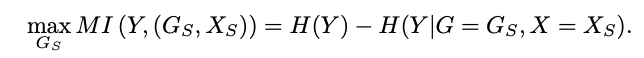Besides, there is a secondary objective: the graph needs to be minimal. Though it was also mentioned in the first objective, we need to have a method to formulate this objective as well. The paper addresses it by adding a loss for the number of edges. Therefore, the loss for GNNExplainer is literally the combination of prediction loss and edge size loss.

#graph #graph-neural-networks #graph-theory #pattern-recognition #machine-learning